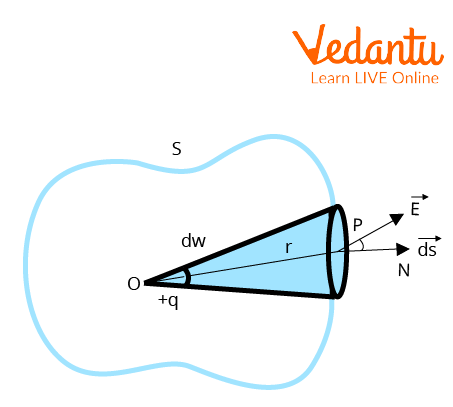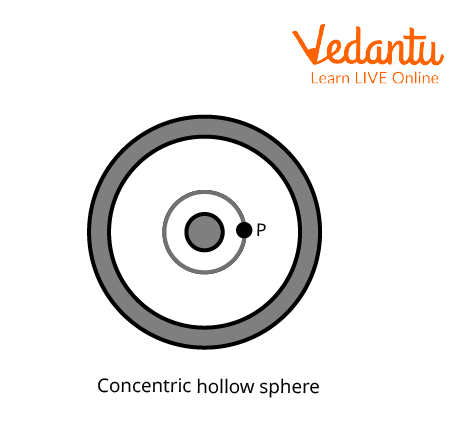Courses
Courses for Kids
Free study material
Offline Centres
More

# Gauss TheoremLast updated date: 24th Nov 2023
Total views: 116.4k
Views today: 2.16k## An Introduction to Gauss Theorem

We are aware that there is always a static electric field around a positive or negative electrical charge and that there is a flow of energy tube or flux inside that static electric field. The electric charge is what radiates or emits this flux. The amount of this flux flow now relies on how much charge is coming from it. Carl Friedrich Gauss developed a theorem to determine this relationship. One of the strongest and most practical theorems in the field of electrical research is this one. This theorem allows us to calculate the flux radiated over the surface area surrounding the charge.

## History of Carl Friedrich Gauss

Initially, Joseph Louis Lagrange (25 January 1736 – 10 April 1813) introduced the concept and later Carl Friedrich Gauss (German mathematician and physicist who is credited with making important advances in branches of science and mathematics) developed the law in the context of determining the attraction force between the ellipsoids.Carl Friedrich Gauss (30 April 1777 – 23 February 1855),

## Gauss Theorem statement

The net charge in the volume encircled by a closed surface directly relates to the net flux through the closed surface.

## Gauss Theorem Formula

The total charge contained within a closed surface is proportional to the total flow contained within it, according to the Gauss theorem. So, if the total flux is $\phi$ and the electric constant is ${ \in _0}$, the total electric charge Q contained by the surface is

$Q = { \in _0}\phi$

$\Rightarrow \phi =\dfrac {Q} {\in_0}$

where Q stands for total charge within the given surface and ${ \in _0}$ is the electric constant.

## Gauss Theorem Proof

Consider a closed surface S with a charge +q located at a point O within it. On the constrained surface, Point P is located at r from Point O. At point P, the electric field's strength will beGauss Theorem Proof

$\vec E = \dfrac{{1}}{{4{\rm{\pi }}{ \in _0}}}\cdot\dfrac{q}{{{r^2}}}$.......(1)

Electric flux flowing across the region ds that surrounds point P,

$d\phi \; = \;\overrightarrow {E\;} \cdot\overrightarrow {ds}$

$\Rightarrow d\phi \; = \;E\cdot ds\;\cos \theta$

where $\theta$ is the angle between E and ds.

Flux crossing the total surface S,

$\int {\mathop \int_s d\phi \;} = \;\int {\mathop \int_sE\cdot d\cos \theta }$ (2)

Using the value of E from equation (1) in equation (2),

$\mathop \int_s d\phi \; = \;\phi$

$\therefore \phi \; = \;\int {\mathop \int_s\dfrac{1}{{4{\rm{\pi }}{ \in _0}}}\;\dfrac{q}{{{r^2}}}}$

$\Rightarrow \phi \; = \dfrac{1}{{4{\rm{\pi }}{ \in _0}}}\;q\;\mathop \int_s \;\dfrac{{ds\cos \theta }}{{{r^2}}}$

$\because \left( \dfrac {{ds}\cos \theta}{r^2}=\omega\right)$

$\Rightarrow \phi ~=\dfrac{1}{4\text{ }\!\!\pi\!\!\text{ }{{\in }_{0}}}~q\cdot \omega$

where $\omega$ is the solid angle. However, in this case, the closed surface S at O has a solid angle equal to $4\pi$, therefore,

$\phi \; = \dfrac{{\;1}}{{4{\rm{\pi }}{ \in _0}}}\cdot q\cdot4{\rm{\pi }}$

$\phi \; = \dfrac{1}{{{ \in _0}}}\cdot{\rm{q}}$

Hence Proved.

## Limitations of Gauss Theorem

• It is only relevant to stationary point charges.

• Gauss’ theorem does not describe every aspect of the field. For instance, it says nothing about how an electrostatic field will exert a force on a charge.

• It only applies in situations where the inverse square law is followed.

## Application of Gauss Theorem

Gauss law is majorly applicable in the following situations:

• A uniformly charged infinite straight wire/line charge produces an electric field.

• A uniformly charged infinite plate sheet produces an electric field.

• A uniformly charged thin spherical shell that produces an electric field.

## Solved Examples

1. Find the electric field from the centre of a 6.0 m circle with a 0.2 C charge using the Gauss theorem.

Ans: Given,

$Q = \;0.2$

$r\; = \;0.6m$

By Gauss' law,

$\phi \; = \;\oint \vec E\cdot\vec dA\; = \dfrac{{\;q}}{{{ \in _0}}}$

$E\; = \dfrac{{\;Q}}{{4\pi {r^2}{ \in _0}}}$

$\Rightarrow E\; = \dfrac{{0.2}}{{4 \times 3.14 \times {{\left( {0.6} \right)}^2} \times 8.85 \times {{10}^{ - 12}}}}\; = \;4.99N{C^{ - 1}}$

2. In the X-Y plane is a massive plane charge sheet with a surface charge density of $2.0\; \times {10^{ - 6}}\;C{m^{ - 2}}$. Find the electric field's flux through a circle with a radius of 1 cm that is entirely within the positive x, y, and z planes and whose normal forms a 60-degree angle with the Z-axis.

Ans: Given,

$\sigma \; = \;2.0\; \times {10^{ - 6}}\;C{m^{ - 2}}$

$\theta \; = \;{60^ \circ }\\r\; = \;1\;cm$

The electric field $E\; = \;\sigma /2{ \in _0}$ is in the direction away from the planar charge sheet. The field is along the Z-axis at the indicated location.

Gauss's theorem states that the flux

$\overrightarrow {E\cdot} \delta \vec s\; = \;E\cdot\delta s\;\cos \theta$

$\Rightarrow E\cdot\delta s\;\cos \theta \; = \dfrac{{\;\sigma }}{{2{ \in _0}}} \times \pi {r^2}\cos {60^\circ }$

$\Rightarrow \dfrac{{\;{\rm{\sigma }}}}{{2{ \in _0}}} \times \pi {r^2}\cos {60^\circ }\; = \dfrac{{\;2.0 \times {{10}^{ - 6}}C{m^{ - 2}}}}{{2 \times 8.85 \times {{10}^{ - 12}}{{\rm{C}}^2}N{m^2}}} \times 3.14 \times {10^{ - 4}}{{\rm{m}}^2} \times \dfrac{1}{2}$

$\Rightarrow {\rm{E}}\;\; = \;17.5N{m^2}{{\rm{C}}^{ - 1}}$

3. A sphere with a radius of 1 cm has an evenly distributed charge of $4 \times {10^8}$ C. A concentric, hollow conducting sphere with a 7 cm radius surrounds it. Calculate the electric field at a point 2 cm from the centre.

Ans: Let's say we need to locate the field at point P. P should be traversed by a concentric spherical surface. Since every point on this surface is equal, the field at every one of them will have the same magnitude and be radial in direction due to symmetry.Concentric Hollow Sphere

This surface's flux through it,

$\oint \overrightarrow {E\cdot} \vec ds\; = \;\oint EdS\; = \;E\oint dS$

$E\oint dS\; = \;4\pi {r^2}{\rm{E}}$

According to the Gauss law, this flux equals the charge q that is present inside the surface divided by ${ \in _0}$. Thus,

$4\pi {r^2}{\rm{E}}\; = \dfrac{{\;{\rm{q}}}}{{{ \in _0}}}$

$\Rightarrow {\rm{E}}\; = \dfrac{{\;{\rm{q}}}}{{4\pi {r^2}{ \in _0}}}$

$\Rightarrow {\rm{E}}\; = \;9 \times {10^9} \times \left[ {\dfrac{{\left( {\;4 \times {{10}^{ - 8}}} \right)}}{{\;4 \times {{10}^{ - 4}}}}} \right]$

${\rm{E}}\; = \;9 \times {10^5}N{C^{ - 1}}$

## Important Formulas to Remember from Gauss Theorem

• The general formula for the Gauss theorem is

$Q = { \in _0}\phi$

$\Rightarrow \phi =\dfrac{ Q} {\in_0}$

• Due to Infinite Wire, electric Field E is

$E = \sigma /2\pi { \in _0}$

• Near the plane's charge sheet, the electric field is

$E\; = \dfrac{{\;\sigma }}{{2\pi r{ \in _0}}}$

$E\; = \dfrac{{\;kq}}{{{r^2}}}$

## Conclusion

The Gauss theorem correlates the charges present on the enclosed surface to the 'flow' of electric field lines. The net electric flow is always zero if no charges are contained by a surface. Accordingly, the quantity of electric field lines entering the surface equals the quantity leaving the surface.

## FAQs on Gauss Theorem

1. What does the Gauss Divergence Theorem mean?

The "Gauss Divergence Theorem" is the most crucial theorem in calculus. Numerous challenging integral problems are solved using this theory. It contrasts the volume integral and the surface integral. It means that it explains how the two are related. The Gauss Divergence Theorem states that the volume integral of the divergence of a vector field A over the volume (V) covered by a closed surface is equal to the surface integral of a vector field A over a closed surface.

2. What does Gauss's Theorem look like in differential form?

The net-outward normal electric flux through any closed surface of any shape is equal to $1/{ \in _0}$ times the entire amount of charge held within that surface, according to Gauss's theorem.

The electric field and the charge distribution at a specific location in space are related by Gauss' law in its differential version. To clarify, according to the law, the volume charge density $\rho$ at a specific position will equal to the electric field's divergence E at that location. It is displayed as:

$\Delta E = \dfrac{\rho} { \in _0}$

3. Define linear, surface, and volume charge density.

The linear charge density $(\lambda )$ at any point on a line charge distribution is the amount of charge per unit length, expressed in coulombs per metre $c{m^{ - 1}}$.

Surface charge density is a measure of how much electric charge has accumulated in a given field. The dimension helps determine the overall quantity of electric charge based on the provided dimension, even if it may refer to the area, length, or volume of the electrical body.

Volume Charge density describes how much charge is contained in the given volume. $\rho$ can be used to indicate an electric charge.